Refer to our Texas Go Math Grade 8 Answer Key Pdf to score good marks in the exams. Test yourself by practicing the problems from Texas Go Math Grade 8 Unit 5 Study Guide Review Answer Key.

Module 12 Transformations and Congruence

Perform the transformation shown. (Lessors 12.1, 12.2, 12.3)

Question 1.
Reflection over the x-axis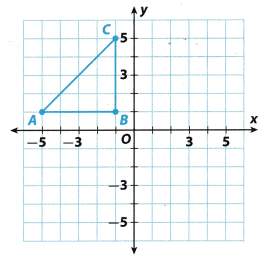In this task, we are going to Reflect triangle with given vertices over x-axis.

Reflection is shown in equation (x, y) → (x, y).
Coordinates after reflection
A(-5, 1) → A’-5, 1) …………… (1)
B(-1, 1) → B'( 1, 1) ………….. (2)
C(-1, 5) → C'(- 1, 5) ………….. (3)
Transformations is shown on graph below: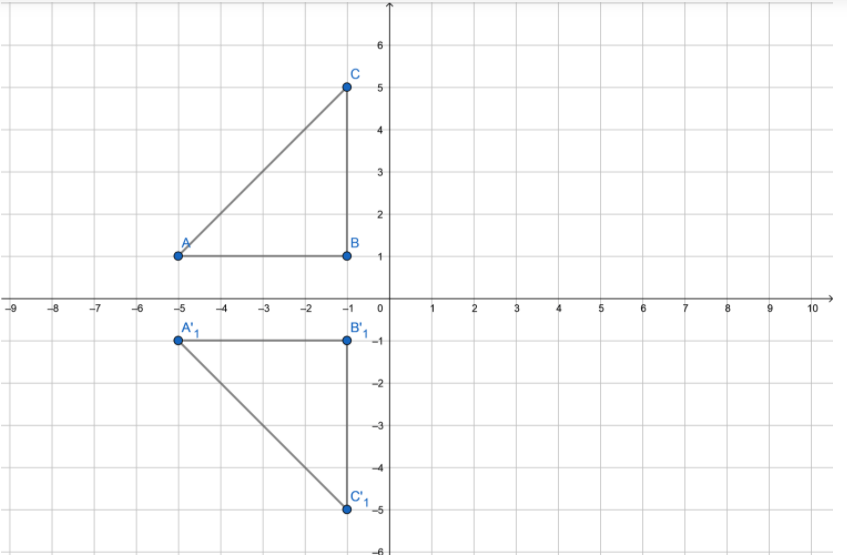Translation 5 units right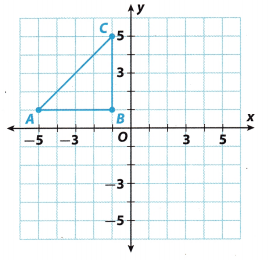In this task, we will translate the given triangle 5 units right

Translation 5 units right is shown by formula (x, y) → (x + 5, y).
Coordinates after refLection
A(-5, 1) → A'(- 5 + 5, 1) → A'(0, 1) ……………. (1)
B(-1, 1) → B'(-1 + 5, 1) → B'(4, 1) …………….. (2)
C(-1, 5) → C'(-1 + 5, 5) → C'(4, 5) ……………. (3)
Transformation is shown in the graph below: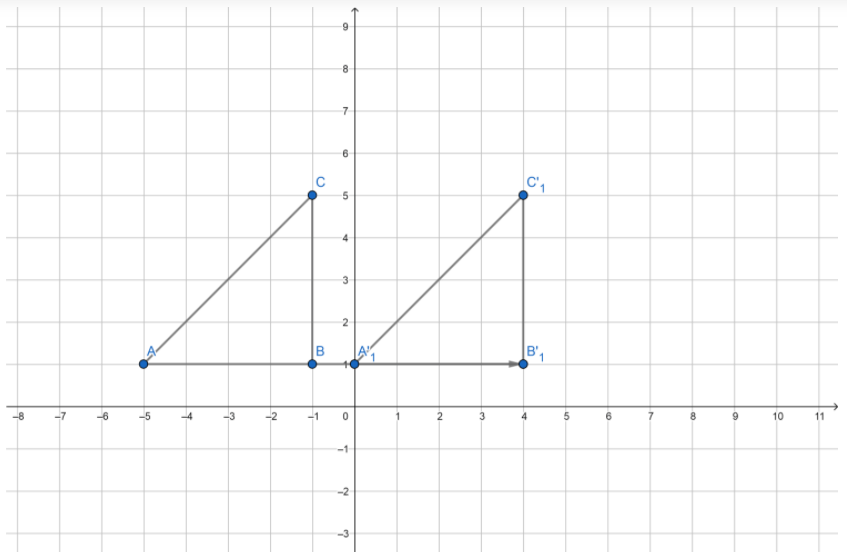Question 3.
Rotation 90° counterclockwise about the origin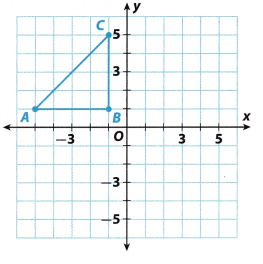The triangle rotates around the origin for 90°. We need to find the coordinates of the image.

Rotation for 90° is shown by transformation (x, y) → ( y, x).
Coordinates after refLection
A(-5, 1) → A'(-1, -5) ……………… (1)
B(-1, 1) → B'(-1, -1) ………………. (2)
C(-1, 5) → C'(-5, -1) ……………… (3)
Rotation is shown on the graph below:Unit 5 End of Unit Assessment Answer Key Grade 8 Question 4.
Translation 4 units right and 4 units down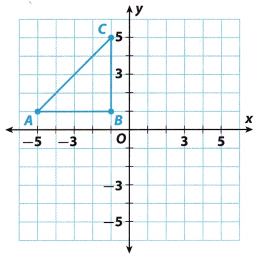We need to translate the given triangle in two steps: The first translation is for Li units right: (x, y) → (x + 4, y), second translation is 4 units down (x, y) → (x, y – 4).

Coordinates after transformation
A(-5, 1) → A'(- 5 + 4, 1) → A'(- 1, 1) → A”(-1, -1, -4) → A”(- 1, -5) ………………….. (1)
B(-1, 1) → B'(- 1 + 4, 1) → B'(3, 1) → B”(3, 1 – 4) → B”(3, -3) ………………. (2)
C(-1, 5) → C'(- 1 + 4, 5) → C'(3, 5) → C”(3, 5 – 4) → C”(3, 1) ………………… (3)

Question 5.
Quadrilateral ABCD with vertices 4(4, 4), B(5, 1), C(5, -1) and D(4, -2) is translated left 2 units and down 3 units. Graph the preimage and the image. (Lesson 12.4)Considering the fact that the figure is translated 2 units to the left and 3 units down, we can calculate the coordinates of the translated image by subtracting 2 from the x coordinate and 3 from the y coordinate.

Therefore, using my explained principle, we can calculate the coordinates of the translated image as follows:
A(4, 4) ⇒ A'(2, 1)
B(5, 1) ⇒ B'(3, -2)
C(5, 1) ⇒ C'(3, -4)
D(4, 2) ⇒ D'(2, -5)
After finding the obtained coordinates on the plane, we can connect them and get the translated image.
The picture below illustrates the preimage and image.Transformation Study Guide Answer Key Unit 5 Math Test 8th Grade Question 6.
Triangle RST has vertices at (-8, 2), (-4, 0), and (-12, 8). Find the vertices after the triangle has been reflected over the y-axis. (Lesson 1 2.4)
Let’s find the coordinates of the given point after reflection over the y-axis. Reflection over the y-axis is shown in the formula
(x, y) → (-x, y).
Coordinates after reflection
A(-8, 2) → A'(8, 2) ………….. (1)
B(-4, 0) → B'(4, 0) …………… (2)
C(-12, 8) → C’12, 8) …………… (3)
Transformation is shown on the graph below.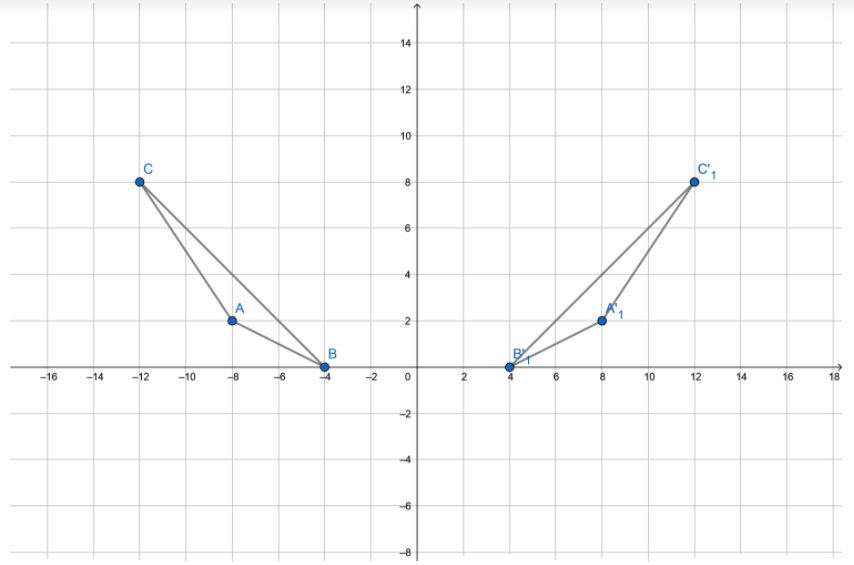Question 7.
Triangle XYZ has vertices at (3, 7), (9, 14), and (12, -1). Find the vertices after the triangle has been rotated 180° about the origin. (Lesson 1 2.4)
The triangle is rotated by 180°. Rotation is given by equation (x, y) → (-x, -y).
Rotated triangle ABC is shown on the graph below: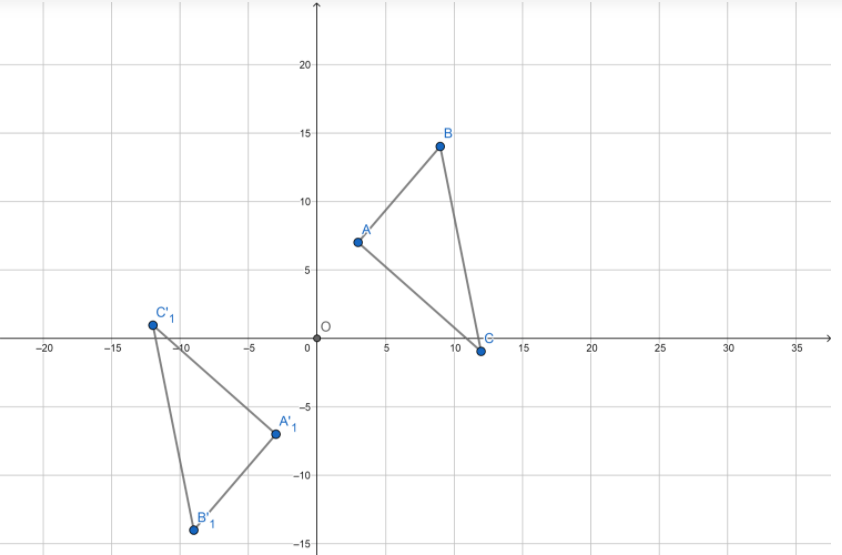Coordinates after rotation are:
X(3, 7) → X'(-3, -7) …………….. (1)
Y(9, 14) → Y'(-9, -14) …………… (2)
Z(12, -1) → Z'(-12, 1) ……………. (3)

Module 13 Dilations, Similarity, and Proportionality

Question 1.
For each pair of corresponding vertices, find the ratio of the x-coordinates and the ratio of they-coordinates. (Lesson 13.1)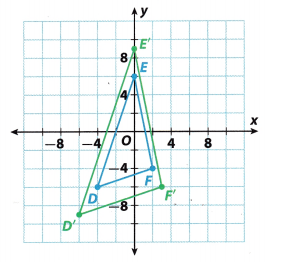Ratio of x-coordinates: ________________
Ratio of y-coordinates: ________________
What is the scale factor of the dilation? _______________
Ratio of x-coordinates: 1.5
Ratio of y-coordinates: 1.5
F'(x,y)/F(x,y) = x/3/2 = y/-6/-4 = 1.5
Thus the scale factor of the dilation is 1.5

Andrew’s old television had a width of 32 inches and a height of 18 inches. His new television is larger by a scale factor of 2.5. Find the perimeter and area of Andrew’s old television and his new television. (Lesson 13.3)
Perimeter of old TV: ________________
Area of old TV: ________________

Perimeter of new TV: ________________
Area of new TV: ________________
The television is in the shape of a rectangle.
Perimeter of the old TV = 2( w + h)
Width = 32 inches.
Height = 18 inches.
= 2(32 + 18)
= 100 square inches.
Area of the old TV = w x h = 32 x 18 = 576.
The perimeter of new TV = perimeter of old TV x 2
= 100 x 2
= 200 square inches
Area of the new TV = area of the old TV x square of 2
= 576 x 4
= 2304.

Dilate each figure with the origin as the center of the dilation. List the vertices of the dilated figure then graph the figure. (Lesson 13.2)

Question 3.
(x, y) → ($$\frac{1}{4}$$x, $$\frac{1}{4}$$y)Firstly, we will write down the coordinates of points X, Y and Z, so we could calculate coordinates after dilation.
Therefore:
X(-8,4)
Y(-4, 4)
Z(4,8)
Using the algebraic expression of dilation (x, y) → ($$\frac{1}{4}$$x, $$\frac{1}{4}$$y), we can calculate the vertices of the dilated figure as following:
X(-8, 4) ⇒ X'(-2, 1)
Y(-4, -4) ⇒ Y'(-1, -1)
Y(4, 8) ⇒ Y'(1, 2)
We can graph obtained coordinates and connect them to get the preimage and image. Picture below shows the figure before and after dilation.(x, y) → (2x, 2y)Rectangle WXYZ has vertices at ( 2, 1), (- 2, 1), (2, -1), and (2, 1). It is first dilated by (x, y) → (2x, 2y), and then translated by (x, y) → (x, y + 3). Let us determine the vertices of the image.
a. Determining the vertices by dilating the image from (x, y) → (2x, 2y). using the points (-2, -1), (-2, 1), (2, -1), and (2, 1).
(-2, -1) → (-4, -2)
(-2, 1) → (-4, 2)
(2, -1) → (4, -2)
(2, 1) → (4, 2)
Translating the ¡mage from (x, y) → (x, y + 3), using the points (-4, -2), (-4, 2), (4, -2), and (4, 4)
(-4, -2) → ( 4, 1)
(-4, 2) → (-4, 5)
(4, -2) → (4, 1)
(4, 2) → (4, 5)
Thus, the vertices of the image are (-4, 1), (-4, 5), (4, 1), and (4, 5).

b. Two figures are said to be congruent if one can be obtained from the other by a sequence of translations, reflections, and rotations. Congruent figures have the same size and shape. Now, Let us determine if the preimage and the translated image are congruent and/or similar.
Let us illustrate the images using the graph.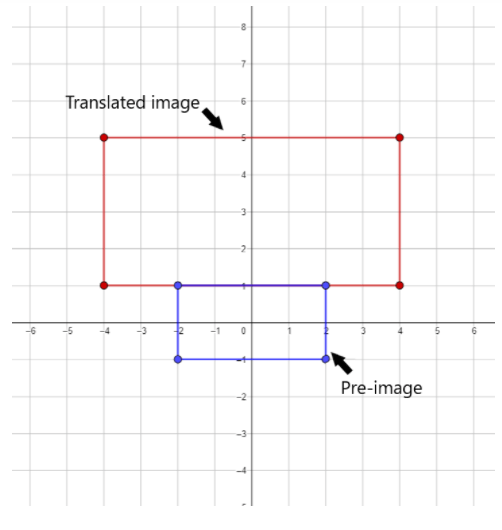As we can see on the graph the two images are both similar in shape but their sizes are different, the translated image is obviously bigger than the image, so the pre image and translated image are similar but not congruent.

Question 1.
CAREERS IN MATH Contractor Fernando is expanding his dog’s play yard. The original yard has a fence represented by rectangle LMNO on the coordinate plane. Fernando hires a contractor to construct a new fence that should enclose 6 times as much area as the current fence. The shape of the fence must remain the same. The contractor constructs the fence shown by rectangle L’M’N’O’.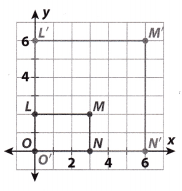a. Did the contractor increase the area by the amount Fernando wanted? Explain.
Answer: Yes, the original area was 6 square units and the new area is 36 square units.
Explanation:
Area of LMNO,
A = 3 × 2 = 6
Area of rectangle LMNO is 6 square units
Area of L’M’N’O’,
A = 6 × 6 = 36
Area of rectangle L’M’N’O is 36 square units

b. Does the new fence maintain the shape of the old fence? Flow do you know?
Answer: No, the corresponding side lengths are not proportional.

Unit 5 Math Test 8th Grade Study Guide Answer Key Question 2.
A sail for a sailboat is represented by a triangle on the coordinate plane with vertices (0, 0), (5, 0), and (5, 4). The triangle is dilated by a scale factor of 1.5 with the origin as the center of dilation. Find the coordinates of the dilated triangle. Are the triangles similar? Explain.
A sail for a sailboat is represented by a triangle on the coordinate plane with vertices (0, 0), (5, 0), and (5, 4). The triangle is dilated by a scale factor of 1.5 with the origin as the center of dilation. To determine the vertices of the dilated triangle, let us use the expression below
(x, y) → (1.5x, 1.5y)
Having the vertices of the triangle (0, 0), (5, 0), and (5, 4), the dilated triangle has the vertices which are
(x, y) → (1.5x, 1.5y)
(0, 0) → (1.5(0), 1.5(0)) = (0, 0)
(5, 0) → (1.5(5), 1.5(0)) = (7.5, 0)
(5, 4) → (1.5(5), 1.5(4)) = (7.5, 6)
Thus, the vertices of the dilated triangle are (0, 0), (7.5, 0), and (7.5, 6).
Looking at the graph of the two figures, we can say that they are similar triangles since they have the same shape and their corresponding angles are congruent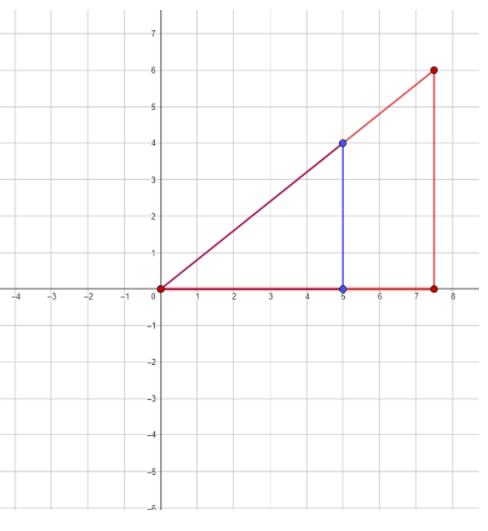Texas Go Math Grade 8 Unit 5 Mixed Review Texas Test Prep Answer Key

Selected Response

Question 1.
What would be the orientation of the figure below after a reflection over the x-axis?(B)

Explanation:
Lets find the picture that corresponds to given figure.Question 2.
A triangle with coordinates (4, 2), (0, 3), and (-5, 3) is translated 5 units right and rotated 180° about the origin. What are the coordinates of its image?
(A) (9, 2), (-1, -2), (5, -7)
(B) (-10, 3), (-1, 2), (-5, -3)
(C) (2,-1), (-3, -5), (3, -10)
(D) (-9, -2), (-5, 3), (0, -3)
(D) (-9, -2), (-5, -3), (0, -3)

Explanation:
Given triangle is translated 5 units right, and rotated by 18O. Let’s find coordinates after the transformation.
Translation 5 units right is shown by equation (x, y) → (x + 5, y) Rotation by 180° is (x, y) → (- x, y)Coordinates after rotation
A(4, 2) → A'(4 + 5, 2) → A'(9, 2) → A”(-9, -2) ……………………… (1)
B(0, 3) → B'(0 + 5, 3) → B'(5, 3) → B”(- 5, -3) ………………… (2)
C(-5, 3) → C'(-5 + 5, 3) → C'(0, 3) → C”(0, -3) ………………… (3)

Texas Go Math Grade 8 Unit 5 End of Unit Assessment Answer Key Question 3.
Quadrilateral LMNP has sides measuring 16, 28,12, and 32. Which could be the side lengths of a dilation of LMNP?
(A) 24, 40, 18, 90
(B) 32, 60, 24, 65
(C) 20, 35, 15, 40
(D) 40, 70, 30, 75
(C) 20, 35, 15, 40

Explanation:
Quadrilateral LMNP has sides measuring 16, 28, 12, and 32. A quadriLiteral which is dilation of LMNP have proportional side lengths, so we should see if the scale factor is correct.
(A) 24, 40, 18, 90
$$\frac{16}{24}=\frac{2}{3}$$
$$\frac{28}{40}=\frac{7}{10}$$
⇒ $$\frac{2}{3} \neq \frac{7}{10}$$
⇒ This quadrilateral is not dilation of LMNP

(B) 32, 60, 24, 65
$$\frac{16}{32}=\frac{1}{2}$$
$$\frac{28}{60}=\frac{7}{15}$$
⇒ $$\frac{1}{2} \neq \frac{7}{15}$$
⇒ This quadrilateral is not dilation of LMNP

(C) 20, 35, 15, 40
$$\frac{16}{20}=\frac{4}{5}$$
$$\frac{28}{35}=\frac{4}{5}$$
$$\frac{12}{15}=\frac{4}{5}$$
$$\frac{32}{40}=\frac{4}{5}$$
⇒ This quadrilateral is a dilation of LMNP

(D) 40, 70, 30, 75
$$\frac{16}{40}=\frac{2}{5}$$
$$\frac{28}{70}=\frac{2}{5}$$
$$\frac{12}{30}=\frac{2}{5}$$
$$\frac{32}{40}=\frac{4}{5}$$
⇒ $$\frac{2}{5} \neq \frac{4}{5}$$
⇒ This quadrilateral is dilation of LMNP

Question 4.
The table below represents which equation?(A) y = x + 2
(B) y = -x
(C) y = 3x + 6
(D) y = -3x – 2
(D) y = -3x – 2

Explanation:
Lets write linear equation using values from the table, and change in equation y = kx + n to calculate coefficients k and n.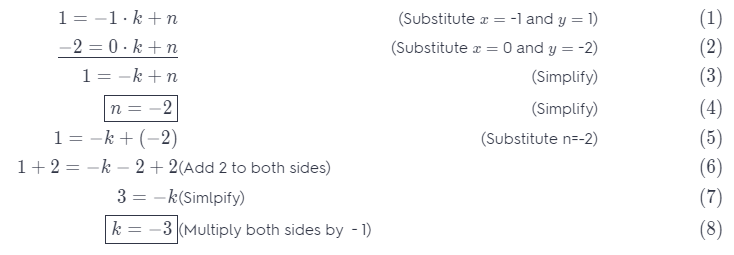Using k = -3 and n = -2 we can now write the linear equation.
y = -3x – 2

Question 5.
Which of the following is not true of a trapezoid that has been translated 8 units down?
(A) The new trapezoid is the same size as the original trapezoid.
(B) The new trapezoid is the same shape as the original trapezoid.
(C) The new trapezoid is in the same orientation as the original trapezoid.
(D) The y-coordinates of the new trapezoid are the same as the y-coordinates of the original trapezoid.
(D) The y-coordinates of the new trapezoid are the same as the y-coordinates of the original trapezoid.

Explanation:
To determine among the options is not true of a trapezoid that has been translated 8 units down. Let us analyze each statements.

First, the statement is The new trapezoid is the same size as the original trapezoid. It is true because even if it is translated 8 units down, still the new trapezoid has the same size as the original and nothing will be change when it comes to size.

Second, the statement is The new trapezoid is the same shape as the original trapezoid. This statement is correct, because nothing will change in their shape. The new trapezoid is the same as the shape of the original trapezoid.

Third, the statement is The new trapezoid is in the same orientation as the original trapezoid. This is also correct since the direction of the new and original trapezoid remains the same.

Lastly, the statement is The y-coordinates of the new trapezoid are the same as the y-coordinates of the original trapezoid. This statement is not true., since it is said that the trapezoid has been translated 8 units down. The direction of the units is downward which is vertical, then the y-coordinates will change. So, the y-coordinates of the new trapezoid are the not same as the y-coordinates of the original trapezoid.

Question 6.
Which represents a reduction?
(A) (x, y) → (0.9x, 0.9y)
(B) (x, y) → (1.4x, 1.4y)
(C) (x, y) → (0.7x, 0.3y)
(D) (x, y) → (2.5x, 2.5y)
(A) (x, y) → (0.9x, 0.9y)

Explanation:
A dilation can produce a larger figure (an enlargement) or a smaller figure (a reduction). The scale factor k describes how much the figure is enlarged or reduced. If the scale factor k is between 0 and 1 the image is a reduction. If the scale factor is greater than 1, the image is an enlargement.

To determine which represents a reduction among the following, let us identify the scale factor of each given.
(x, y) → (0.9x, 0.9y)
(x, y) → (1.4x, 1.4y)
(x, y) → (0.7x, 0.3y)
(x, y) → (2.5x, 2.5y)
Determining g the scale factor k.
(x, y) → (0.9x, 0.9y); since k = 0.9 is between 0 and 1, then this is reduction
(x, y) → (1.4x, 1.4y); since k = 1.4 is greater than 1, then this is enlargement
(x, y) → (0.7x, 0.3y); cannot be identify as reduction or enlargement since k are different
(x, y) → (2.5x, 2.5y); since k = 2.5 is greater than 1, then this is enlargement
Since (x, y) → (0.9x, 0.9y) represents reduction, then the answer is Letter A.

Question 7.
Grain is stored in cylindrical structures called silos. Which is the best estimate for the volume of a silo with a diameter of 12.3 feet and a height of 25 feet?
(A) 450 cubic feet
(B) 900 cubic feet
(C) 2970 cubic feet
(D) 10,800 cubic feet
Given,
Grain is stored in cylindrical structures called silos.
Diameter = 123 ft
height = 25 ft
The volume of any regular-shaped object = Area of base × height.
The volume of the cylinder = πr²h
V = 3.14 × (12.3/2)² × 25
V = 2970.5 cubic ft
Thus the correct answer is option C.

Question 8.
A rectangle has vertices (8, 6), (4, 6), (8, -4), and (4, -4). What are the coordinates after dilating from the origin by a scale factor of 1.5?
(A) (9, 6), (3, 6), (9, -3), (3, -3)
(B) (10, 8), (5, 8), (10, -5), (5, -5)
(C) (16, 12), (8, 12), (16, -8), (8, -8)
(D) (12, 9), (6, 9), (12, -6), (6, -6)
(D) (12, 9), (6, 9), (12, -6), (6, -6)

Explanation:
A rectangle has vertices (8, 6), (4, 6), (8, 4), and (4, 4). We need to find the coordinates after dilating from the origin by a scare factor of 1.5
Step2 2of3
(8, 6) → 8 ∙ 1.5, 6 ∙ 1.5) → (12, 9) ………….. (1)
(4, 6) → 4 ∙ 1.5, 6 ∙ 1.5) → (6, 9) …………… (2)
(8, -4) → 8 ∙ 1.5, -4 ∙ 1.5) → (12, -6) …………… (3)
(4, 4) → 4 ∙ 1.5, 4 ∙ 1.5) → (6, -6) …………… (4)

Question 9.
Two sides of a right triangle have lengths of 56 centimeters and 65 centimeters. The third side is not the hypotenuse. How long is the third side?
(A) 9 centimeters
(B) 27 centimeters
(C) 33 centimeters
(D) 86 centimeters
Explanation:
Given,
Two sides of a right triangle have lengths of 56 centimeters and 65 centimeters.
The third side is not the hypotenuse.
56² + b² = 65²
b² = 1089
b = 33
Thus the correct answer is option C.

Which statement is false?
(A) No integers are irrational numbers.
(B) All whole numbers are integers.
(C) No real numbers are rational numbers.
(D) All integers greater than or equal to 0 are whole numbers.
(C) No real numbers are rational numbers.

Explanation:
To determine the false statement, let us analyze each statement in the given choices.

The first is “No integers are irrational numbers”. Integers is the set of rational numbers that include zero, positive, and negative numbers. By definition of integers, we can say that all integers are rational numbers and not irrational. Thus, the statement is true.

Second, “All whole numbers are integers”. Whole numbers is the set of zero and natural numbers, and natural numbers are the numbers that include positive integers starting from 1 up to infinity. By definition of whole numbers, we can say that all whole numbers are integers. Thus, the statement is true

Third, “No real numbers are rational numbers.” The real numbers is the set of numbers containing all the rational and all of the irrational numbers. By definition of real numbers, we can say that real numbers can be irrational numbers which contradicts the given statement. Thus, the statement is false.

Fourth. “All integers greater than or equal to 0 are whole numbers”. Whole numbers are the set of zero and natural numbers, and natural numbers are the numbers that include positive integers starting from 1 up to infinity. By definition of whole numbers, we can say that all integers greater than or equal to 0 are whole numbers. Thus, the statement is true

Thus, the false statement among the four choices is the letter C, No real numbers are rational numbers.

Hot Tips! Make sure you look at all answer choices before making your decision. Try substituting each answer choice into the problem if you are unsure of the answer.

Question 11.
Which inequality represents the solution to 1.5x + 4.5 < 2.75x – 5.5?
(A) x > 8
(B) x < 8
(C) x > 12.5
(D) x < 12.5
1.5x + 4.5 < 2.75x – 5.5
10 < 1.25x
8 < x
x >8
Thus the correct answer is option A.

Gridded Response

Question 12.
In what quadrant would the triangle below be located after a rotation of 90° counterclockwise?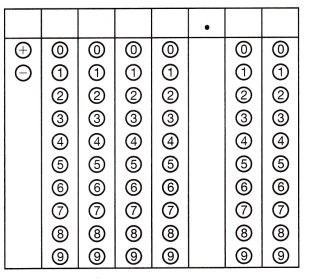The coordinates of the given triangle is (5,-2),(1,-2), (3,-4)
Rotation in 90 degrees counterclockwise about the origin is (x,y) = (-y.x)
(5,-2) = (2,5)
(1,2) = (2, 1)
(3, -4) = (4,3)
After rotation of 90 degrees the triangle is in the 1st quadrant.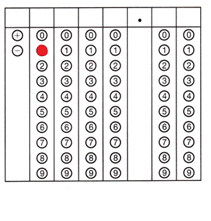Question 13.
An equilateral triangle has a perimeter of 48 centimeters. If the triangle is dilated by a factor of 0.75, what is the length of each side of the new triangle?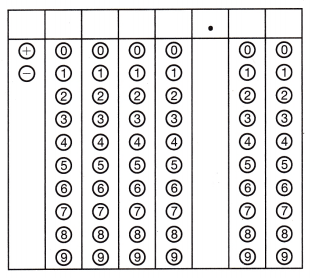The length of each side is 28 cm.
The perimeter of the equilateral triangle is 48 cm.
To find each side of the original triangle, you do 48/3=16cm.
Since the triangle dilates by 0.75,
0.75×16=12cm.
Then you have to add 12 to 16 because the triangle gets bigger by 12 cm.
so 16+12=28 cm.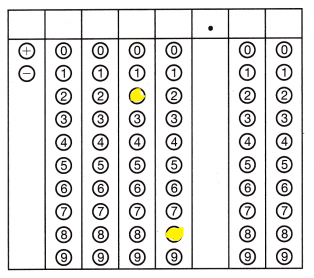Texas Go Math Grade 8 Unit 5 Vocabulary Preview

Use the puzzle to preview key vocabulary from this unit. Unscramble the circled letters within found words to answer the riddle at the bottom of the page.The input of a transformation. (Lesson 12.1)
A transformation that flips a figure across a line. (Lesson 12.2)
A transformation that slides a figure along a straight line. (Lesson 12.1)
A transformation that turns a figure around a given point. (Lesson 12.3)
The product of a figure is made larger by dilation. (Lesson 13.1)
The product of a figure is made smaller by dilation. (Lesson 13.1)
Scaled replicas that change the size but plot the shape of a figure. (Lesson 13.1)

Question.
What do you call an angle that s broken?